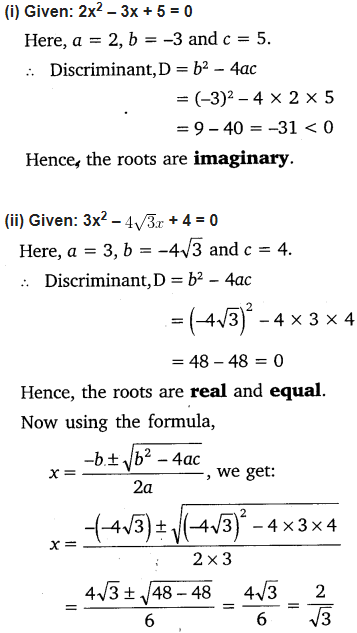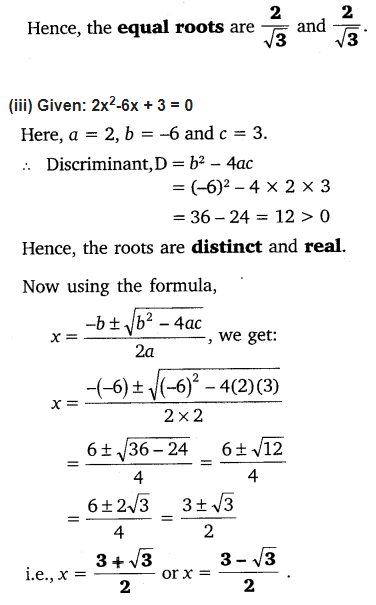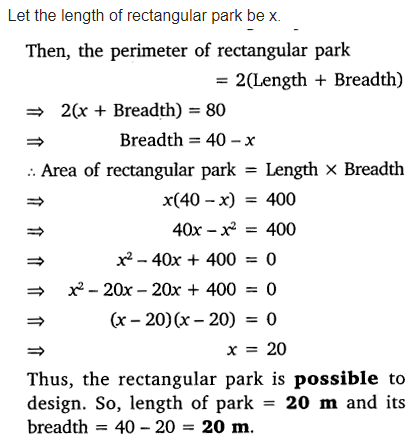# NCERT Solutions For Class 10 Maths Chapter 4 Quadratic Equations Ex 4.4

Get Free NCERT Solutions for Class 10 Maths Chapter 4 Ex 4.4 Quadratic Equations Class 10 Maths NCERT Solutions are extremely helpful while doing homework. Exercise 4.4 Class 10 Maths NCERT Solutions were prepared by Experienced ncert-books.in Teachers. Detailed answers of all the questions in Chapter 4 Maths Class 10 Quadratic Equations Exercise 4.4 Provided in NCERT Textbook

Topics and Sub Topics in Class 10 Maths Chapter 4 Quadratic Equations:

 Section Name Topic Name 4 Quadratic Equations 4.1 Introduction 4.2 Quadratic Equations 4.3 Solution of a Quadratic Equation by Factorisation 4.4 Solution of a Quadratic Equation by Completing the Square 4.5 Nature of Roots 4.6 Summary

You can also download the free PDF of Chapter 4 Ex 4.4 Quadratic Equations NCERT Solutions or save the solution images and take the print out to keep it handy for your exam preparation.

 Board CBSE Textbook NCERT Class Class 10 Subject Maths Chapter Chapter 4 Chapter Name Quadratic Equations Exercise Ex 4.4 Number of Questions Solved 5 Category NCERT Solutions

## NCERT Solutions For Class 10 Maths Chapter 4 Quadratic Equations Ex 4.4

NCERT Solutions for Class 10 Maths Chapter 4 Quadratic Equations Ex 4.4 are part of NCERT Solutions for Class 10 Maths. Here we have given NCERT Solutions for Class 10 Maths Chapter 4 Quadratic Equations Ex 4.4.

Question 1.
Find the nature of the roots of the following quadratic equations. If the real roots exist, find them:
(i) 2x² -3x + 5 = 0
(ii) 3x2 – 4√3x + 4 = 0
(iii) 2x2-6x + 3 = 0
Solution:Question 2.
Find the values of k for each of the following quadratic equations, so that they have two equal roots.
(1) 2x2 + kx + 3 = 0
(2) kx (x – 2) + 6 = 0
Solution:Question 3.
Is it possible to design a rectangular mango grove whose length is twice its breadth, and the area is 800 m2? If so, find its length and breadth.
Solution:Question 4.
Is the following situation possible? If so, determine their present ages.
The sum of the ages of two friends is 20 years. Four years ago, the product of their ages in years was 48.
Solution:Question 5.
Is it possible to design a rectangular park of perimeter 80 m and area 400 m2? If so, find its length and breadth.
Solution:+